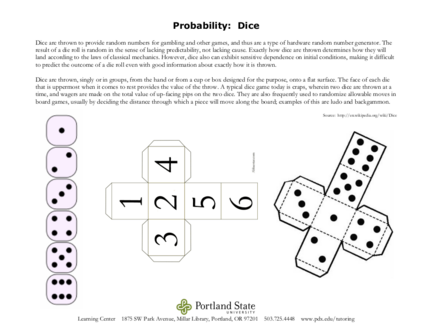# Probability: Case Study of Rolling a pair of diceContributed by:This pdf includes:-
Rolling a Dice
Favorable outcomes
Total outcomes
Probability is the branch of mathematics concerning numerical descriptions of how likely an event is to occur, or how likely it is that a proposition is true. The probability of an event is a number between 0 and 1, where, roughly speaking, 0 indicates the impossibility of the event and 1 indicates certainty.
1. Probability: Dice
Dice are thrown to provide random numbers for gambling and other games, and thus are a type of hardware random number generator. The
result of a die roll is random in the sense of lacking predictability, not lacking cause. Exactly how dice are thrown determines how they will
land according to the laws of classical mechanics. However, dice also can exhibit sensitive dependence on initial conditions, making it difficult
to predict the outcome of a die roll even with good information about exactly how it is thrown.
Dice are thrown, singly or in groups, from the hand or from a cup or box designed for the purpose, onto a flat surface. The face of each die
that is uppermost when it comes to rest provides the value of the throw. A typical dice game today is craps, wherein two dice are thrown at a
time, and wagers are made on the total value of up-facing pips on the two dice. They are also frequently used to randomize allowable moves in
board games, usually by deciding the distance through which a piece will move along the board; examples of this are ludo and backgammon.
Source: http://en.wikipedia.org/wiki/Dice
Learning Center 1875 SW Park Avenue, Millar Library, Portland, OR 97201 503.725.4448 www.pdx.edu/tutoring
2. Probability: Dice
When you roll just one die, there are six different ways the die can land.
When two dice are rolled, there are now 36 different and unique ways the dice can come up. This figure is arrived at by multiplying the
number of ways the first die can come up (six) by the number of ways the second die can come up (six). 6 x 6 = 36.
This graphic shows this very nicely. We've used
two different colored dies to help show a roll of
2-1 is different from a roll of 1-2.
If you use the above graphic and count the
number of times is 6 appears when two dice are
rolled, you will see the answer is eleven. Eleven
times out of 36 or 30.5 %, slightly less than the
33.3% (2/6). When you roll two dice, you have a
30.5 % chance at least one 6 will appear.
This figure can also be figured out
mathematically, without the use of the graphic.
One way to do so is to take the number of ways a
single die will NOT show a 6 when rolled (five)
and multiply this by the number of ways the second die will NOT show a 6 when rolled. (Also five.) 5 x 5 = 25. Subtract this from the total
number of ways two dice can appear (36) and we have our answer...eleven.
Determine the chances of at least one 6 appearing when three dice are rolled.
Take the chances of a 6 NOT appearing on the first die... 5/6
and multiply this by the chances of a 6 NOT appearing on the second die... 5/6 x 5/6 = 25/36
and multiply this by the chances of a 6 NOT appearing on the third die... 25/36 x 5/6 = 125/216
So, there are 125 out of 216 chances of a 6 NOT appearing when three dice are rolled. Simply subtract 125 from 216 which will give us the
chances a 6 WILL appear when three dice are rolled, which is 91. 91 out of 216 or 42.1 %.
Source: Edward D. Collins, http://www.edcollins.com/math-questions.htm
Learning Center 1875 SW Park Avenue, Millar Library, Portland, OR 97201 503.725.4448 www.pdx.edu/tutoring